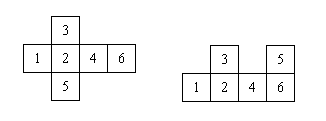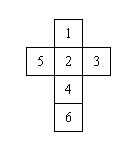### ACSL Practice 2009

Folding six squares connected in some special ways can form a cube. For example, in the diagram below, the six squares on the left can be folded into a cube (with face 1 opposite face 4, face 2 opposite face 6, and face 3 opposite face 5) but the six squares on the right cannot be folded into a cube (faces 3 and 5 overlap).A 6 × 6 array is used to represent the six squares. Only 6 of the 36 cells are used to represent the 6 squares. A cell representing a square contains the number for the square. Other cells contain the number 0. Note that the same 6 squares can be represented in many ways. For example, the invalid six squares of the above example can be represented as follows (the one on the right is obtained after a 90 degree rotation of the one on the left):

```                0 0 0 0 0 0         0 0 0 0 0 0
0 3 0 5 0 0         0 0 0 0 0 0
1 2 4 6 0 0         0 0 0 0 5 6
0 0 0 0 0 0         0 0 0 0 0 4
0 0 0 0 0 0         0 0 0 0 3 2
0 0 0 0 0 0         0 0 0 0 0 1 ```

Given a square representation, determine if the squares can be folded into a cube; if so, find the face opposite face 1.

### Input

The input consists of six lines with each line containing six integers. All but six of the input integers are zeros. The non-zero integers are 1, 2, 3, 4, 5, 6.

### Output

The output file consists of a single integer. The integer is 0 if the squares cannot be folded into a cube; otherwise, the integer is the number of the face opposite face 1.

### Sample Input

```0 0 0 0 0 0
0 0 0 0 0 0
0 0 1 0 0 0
0 5 2 3 0 0
0 0 4 0 0 0
0 0 6 0 0 0```

`4`

### DiagramPoint Value: 20
Time Limit: 2.00s
Memory Limit: 16M